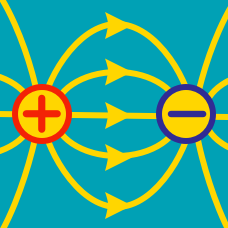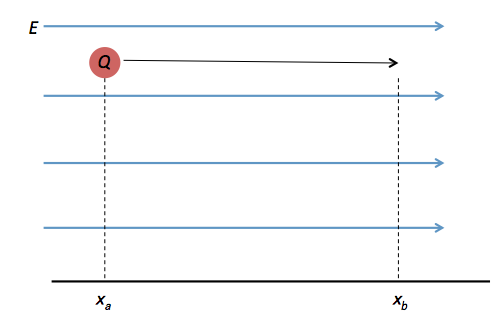Electricity and Magnetism

# Conservative nature of Electric fieldsA constant electric field $E = 5 \text{ V/m}$ exists, as depicted in the above figure. A $Q= 5 \times 10^{-3} \text{ C}$ charge of mass $m = 4 \text{ g},$ initially at rest at $x_a = - 1 \text { m},$ is released. What is its approximate speed at $x_b = 2 \text{ m}?$

A charge of $q = 4.0 \text{ C},$ initially away from a fixed point charge of $1.0 \text{ nC}$ by a distance of $r_1 = 7.0 \text{ m},$ moves closer to a point where the distance is $r_2= 1.0 \text{ m}.$ What is the approximate work done to the charge?

Assume that electric constant is $\epsilon_0 = 8.9 \times 10^{-12} \text{ F/m}.$

Two identical charges with charge $q= 4 \times 10^{-7} \text{ C}$ and mass $m = 3 \text{ g}$ are fixed $r =1.2 \text{ cm}$ apart. Another identical charge is shot from infinity and stops midway between the two. What is its approximate initial speed?

Assume that electric constant is $\epsilon_0 = 8.9 \times 10^{-12} \text{ F/m}.$

A charge of $- 3.0 \times 10^{-3} \text{ C}$ is moved through a potential difference of $3.0 \text{ V}.$ Calculate the work done on the charge.

A $V_b = 12 \text{ V}$ battery is connected to two parallel plates separated by a distance of $d_p = 6 \text{ cm}.$ This creates a uniform electric field between the two plates. If a point charge $Q = 5 \text{ C}$ is put in the middle between the plates, what is the kinetic energy of the charge when it touches the plates?

×